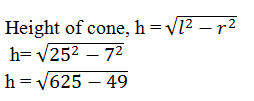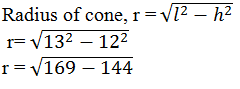Guru

# Find the capacity in litres of a conical vessel with (i) radius 7cm, slant height 25 cm (ii) height 12 cm, slant height 12 cm (Assume π = 22/7) Q.2

• 0

What is the best way for solving the question from class 9th ncert math of Surface areas and Volumes chapter of Ncert of exercise 13.7  of math. What is the best way for solving this question please guide me the best way for solving this question no.2 Find the capacity in litres of a conical vessel with  (i) radius 7cm, slant height 25 cm (ii) height 12 cm, slant height 12 cm  (Assume π = 22/7)

Share

1. (i) Radius of cone, r =7 cm

Slant height of cone, l = 25 cmor h = 24

Height of the cone is 24 cm

Now,

Volume of cone, V = (1/3) πr2h (formula)

V = (1/3)×(22/7) ×72×24

= (154×8)

= 1232

So, the volume of the vessel is 1232 cm3

Therefore, capacity of the conical vessel = (1232/1000) liters (because 1L = 1000 cm3)

= 1.232 Liters.

(ii) Height of cone, h = 12 cm

Slant height of cone, l = 13 cmr = 5

Hence, the radius of cone is 5 cm.

Now, Volume of cone, V = (1/3)πr2h

V = (1/3)×(22/7)×52×12 cm3

= 2200/7

Volume of cone is 2200/7 cm3

Now, Capacity of the conical vessel= 2200/7000 litres (1L = 1000 cm3)

= 11/35 litres

• 0